# SSC Higher Math Assignment 2021 & 2022

SSC Higher Math Assignment 2021 & 2022 Answer. The 5th assignment of Higher Math subject of SSC 8th week has been published. In addition to today’s assignment, SSC exam 2021 & 2022 will have to solve a total of eight assignments in higher mathematics. Although SSC Higher Math’s 8th, 7th, 6th, 5th, 4th and 2nd week assignment, it is the 1st, 2nd, 3rd, 4th, 6th, 7th & 8th assignments in a higher mathematics subject. Today we will discuss how to solve SSC Higher Mathematics Assignment. Also for those examinees who do not understand how to solve the Higher Math, SSC Assignment, I will create a sample solution of Higher Math assignment. And later we will upload the answer or solution of SSC 8th week & 7th week & 6th week & 5th week & 4th week & 2nd week higher math assignment through our website.

So that you can create a solution or answer for your SSC Advanced Mathematics Assignment by looking at our sample answer sheet. But the solution of the assignment cannot be written by looking at our sample answer sheet and copying it completely. Because if it is copied then the same answer can be the solution of many examinees. So it is better to use your creativity and answer without copying.

### SSC Higher Math 8th Week Assignment 2021অ্যাসাইনমেন্ট: ত্রিকোণমিতি সংক্রান্ত সমস্যা সমাধান।

### SSC Higher Math 8th Week Assignment 2021 Answer[Join]

### SSC Higher Math 7th Week Assignment 2022

SSC 2022 7th week Higher Mathematics Assignment has been published. 2022 SSC has been asked to solve several geometric equations in the 7th week of Higher Mathematics Assignment. All the problems that need to be solved as an assignment task are given in the figure. A total of four questions have to be answered as SSC 2022 7th week higher math assignment. The number allotted for the four questions is 14. So the highest marks can be achieved if SSC 2022 can provide the correct solution to all the questions of 7th week Higher Mathematics Assignment.2022 SSC will be able to acquire the concept of Cartesian coordinates in the plane through the 7th week higher mathematics assignment. Will also be able to determine the distance between two points. SSC 2022 will be able to determine the straight line equation if the 7th week higher math assignment is completed smoothly. Also be able to determine the area of ​​a triangle and a quadrilateral through coordinates. Also the subjects that must be mentioned in the answer sheet for solving the Higher Mathematics Assignment of SSC 2022 7th week are given through the diagram.

### SSC Higher Math 7th Week Assignment 2022 Answer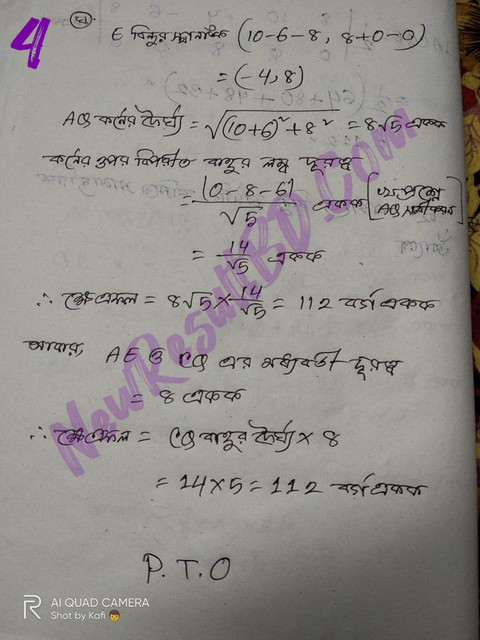[Join]

### SSC Higher Math 6th Week Assignment 2021অ্যাসাইনমেন্ট: অসীম ধারা সংক্রান্ত সমস্যা সমাধান

### SSC Higher Math 6th Week Assignment 2021 Answer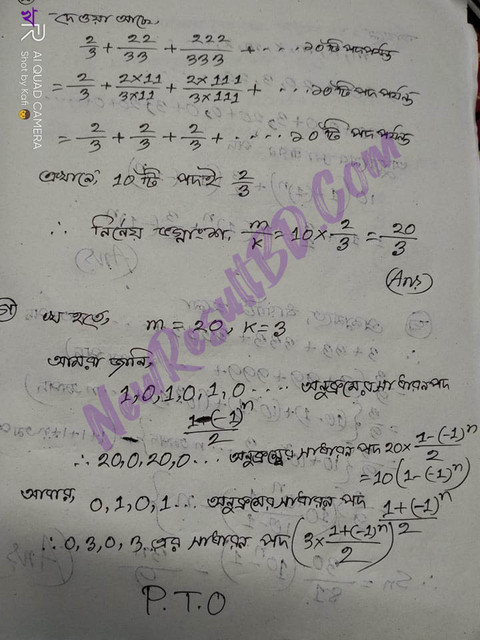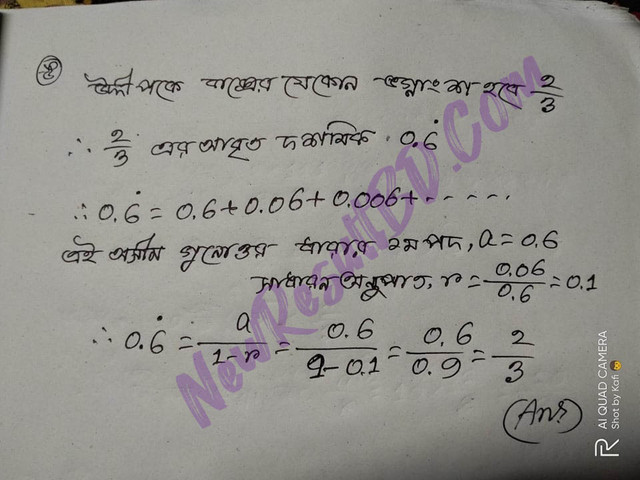[Join]

## SSC Higher Math 5th Week Assignment 2021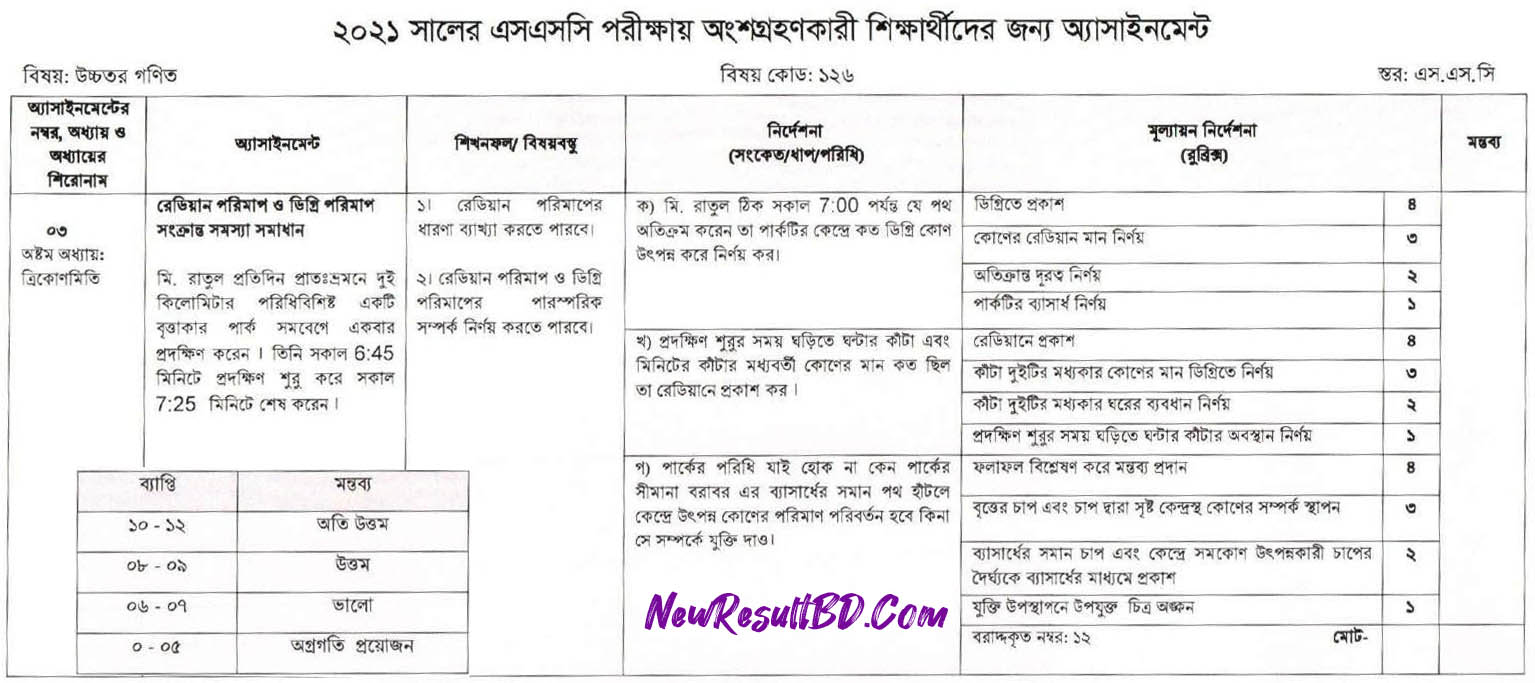অ্যাসাইনমেন্ট: রেডিয়ান পরিমাপ ও ডিগ্রী পরিমাপ সংক্রান্ত সমস্যা সমাধান।

### SSC Higher Math 5th Week Assignment 2021 Answer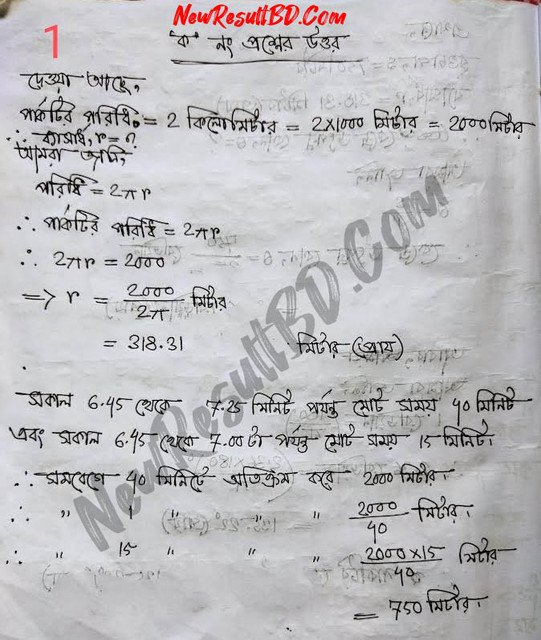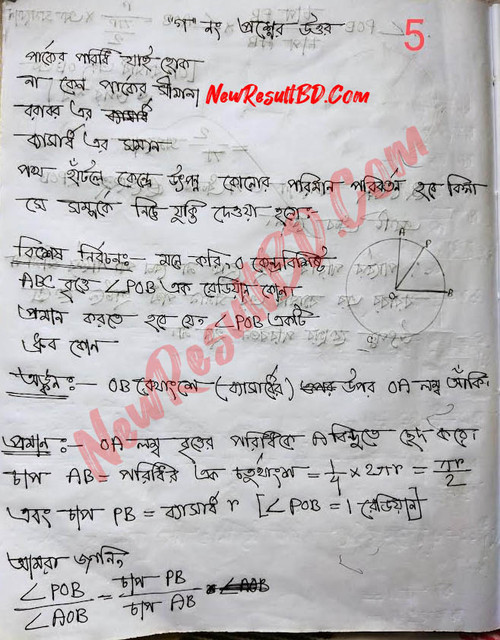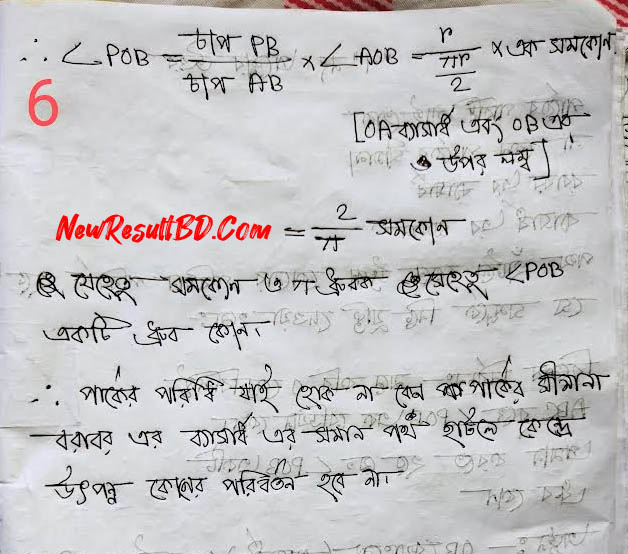[Join]

## SSC Higher Math 4th Week Assignment 2021

I will now talk about how to solve or answer the SSC 4th Week Higher math assignment. Not to mention at the outset, only those SSC candidates who have higher mathematics as a compulsory subject should read our article carefully. And today’s article does not apply to those who have higher mathematics as an optional subject. Why there is no need to solve any type of assignment of an optional subject.The 4th Week of Higher Mathematics Assignments have been selected from Chapter XI. The title of the eleventh chapter is Coordinate Geometry. This is the second assignment of higher mathematics for SSC candidates. The first assignment was given from the mathematical part. But the 4th Week higher math assignment was given from the geometric part. As part of SSC 4th week Higher Mathematics Assignment, it is necessary to solve straight line problems through coordinate geometry. So to solve the problem of straight lines one has to take the help of coordinate geometry.

### SSC Higher Math 4th Week Assignment 2021 Answer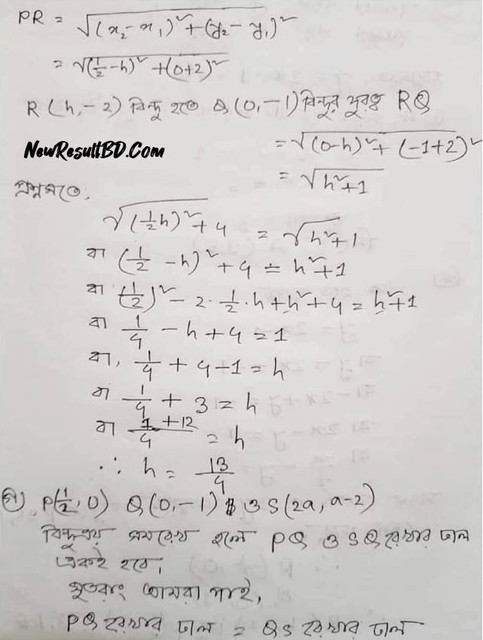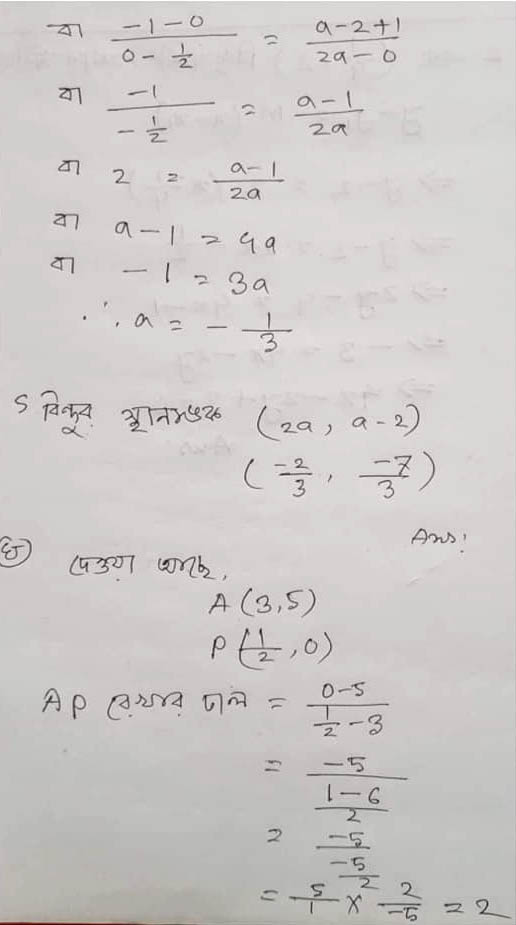There is a lot to learn from the SSC 4th Week higher math assignment. Students who are able to complete the 4th Week higher math assignments correctly should know well about the following topics. For example, one can gain an idea of ​​Cartesian coordinates on a plane. Also, be able to determine the distance between two points. And be able to explain the concept of a straight line slope. Also, be able to accurately determine straight-line equations. Will specialize in determining the area of ​​a triangle. Also, be able to represent straight-line equations with the help of text diagrams.

If the above subjects are well presented, the students will be able to get the highest marks from the 4th Week Higher Mathematics assignment. So all the students who want to get the highest marks from higher mathematics subjects please follow us. Try to solve the SSC 4th Week higher math assignment by following all the instructions we have given.

## SSC Higher Math 2nd Week Assignment 2021

Questions from Chapter XI were first selected as a work of higher mathematics assignment. The subject title of the eleventh chapter of SSC Higher Mathematics is Coordinate Geometry. Here a pentagonal figure is provided as the work of the assignment. Prove that the vertices of the pentagon rotate counterclockwise. Here students need to acquire knowledge about several geometric subjects in order to solve the 2nd week assignment of Higher Mathematics. Such as the concept of coordinates, determining the distance between two points, determining the equation of a straight line, determining the area of ​​a triangle through coordinates as well as drawing geometry related to a quadrilateral. First of all, you have to know the above issues well. Then SSC Higher Mathematics Assignment 2021 will have to be solved.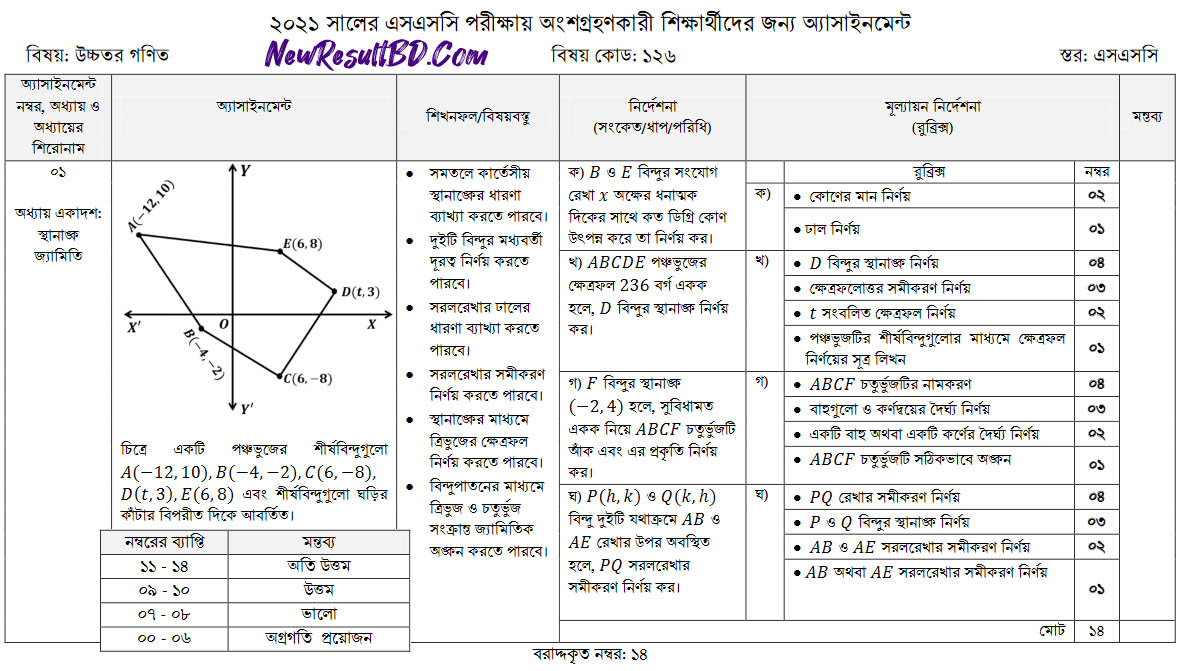## SSC Higher Math Assignment 2021 Answer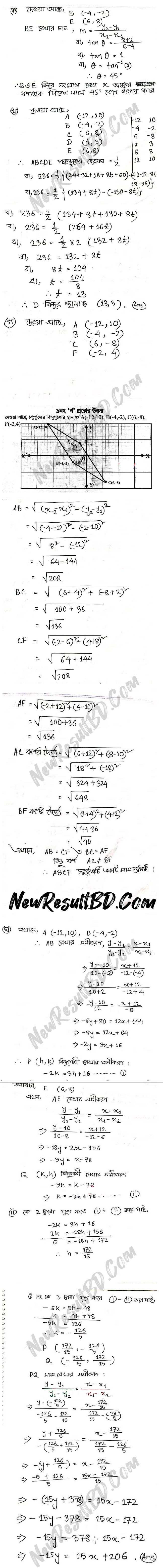[Join]

### How to solve SSC 2nd week Higher Mathematics Assignment?

Some instructions have been given for solving SSC 2nd week and higher math first assignment. Assign the solution to the assignment by following the instructions. Also, some issues must be mentioned in the solution or answer sheet. Otherwise, it will not be possible to get good marks from higher math assignments. The answer sheet must show the value of the angle and the slope. It is provided below:

• The value of the angle and the slope must be determined.
• Find the coordinates of point D.
• The post-field equation has to be determined and shown.
• The area containing T needs to be determined.
• Write the formula for determining the area through the vertices of the pentagon.
• ABCD quadrilateral needs to be named.
• The length of the arms and ears should be determined.
• The ABCD quadrilateral must be drawn correctly.
• Find the equation of the PQ line.
• The coordinates of the points P and Q need to be determined.
• Find the equations AB and AE in a straight line.

### Higher Math 2nd week Assignment Lessons

We can learn a lot if we can complete the 2nd week assignment of higher mathematics properly. For example, we can explain the concept of Cartesian coordinates in the plane. Also, be able to determine the distance between two points. Explain the concept of straight-line slope. Also, be able to determine straight-line equations. I can find the area of ​​a triangle through coordinates. I will also be able to draw geometric figures of triangles and quadrilaterals through point distortion.